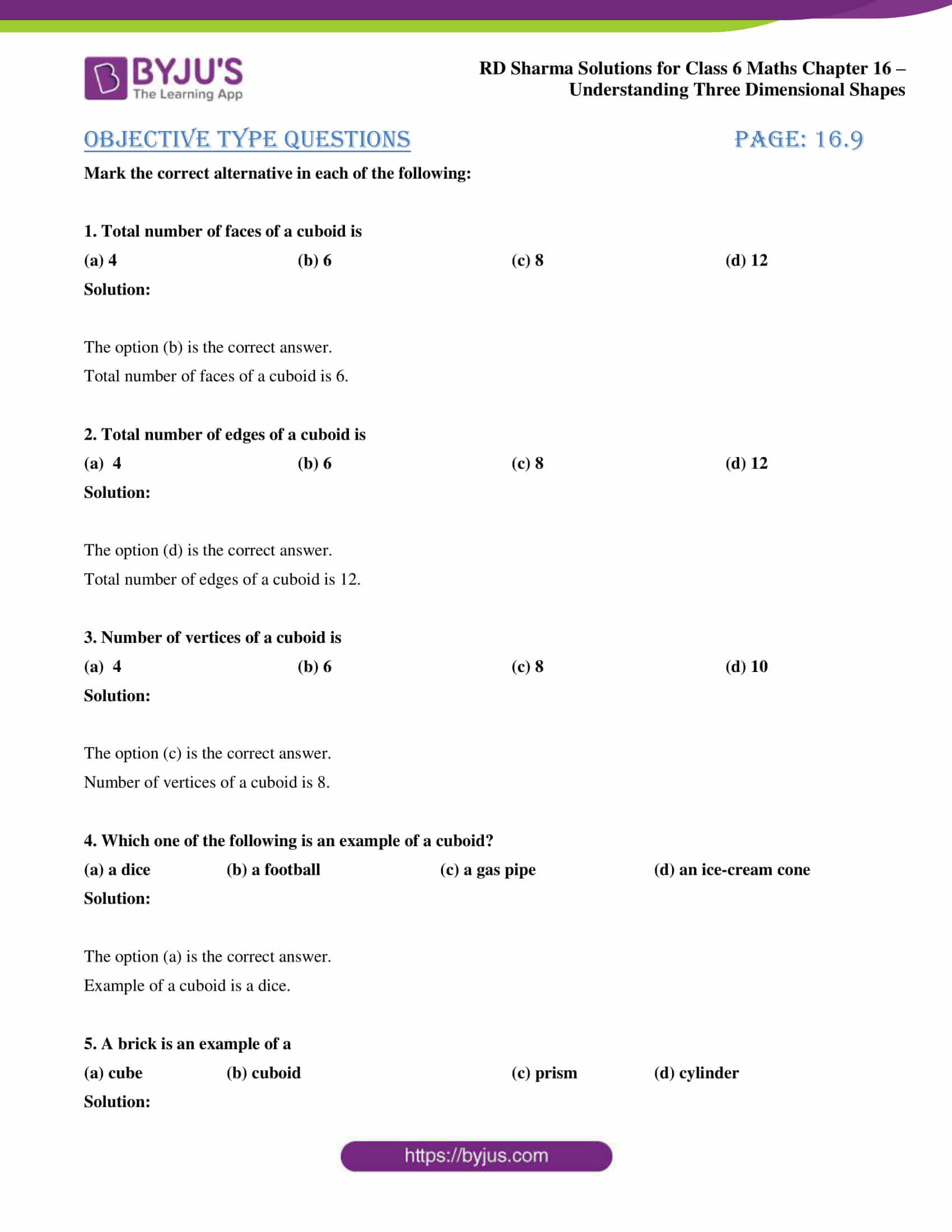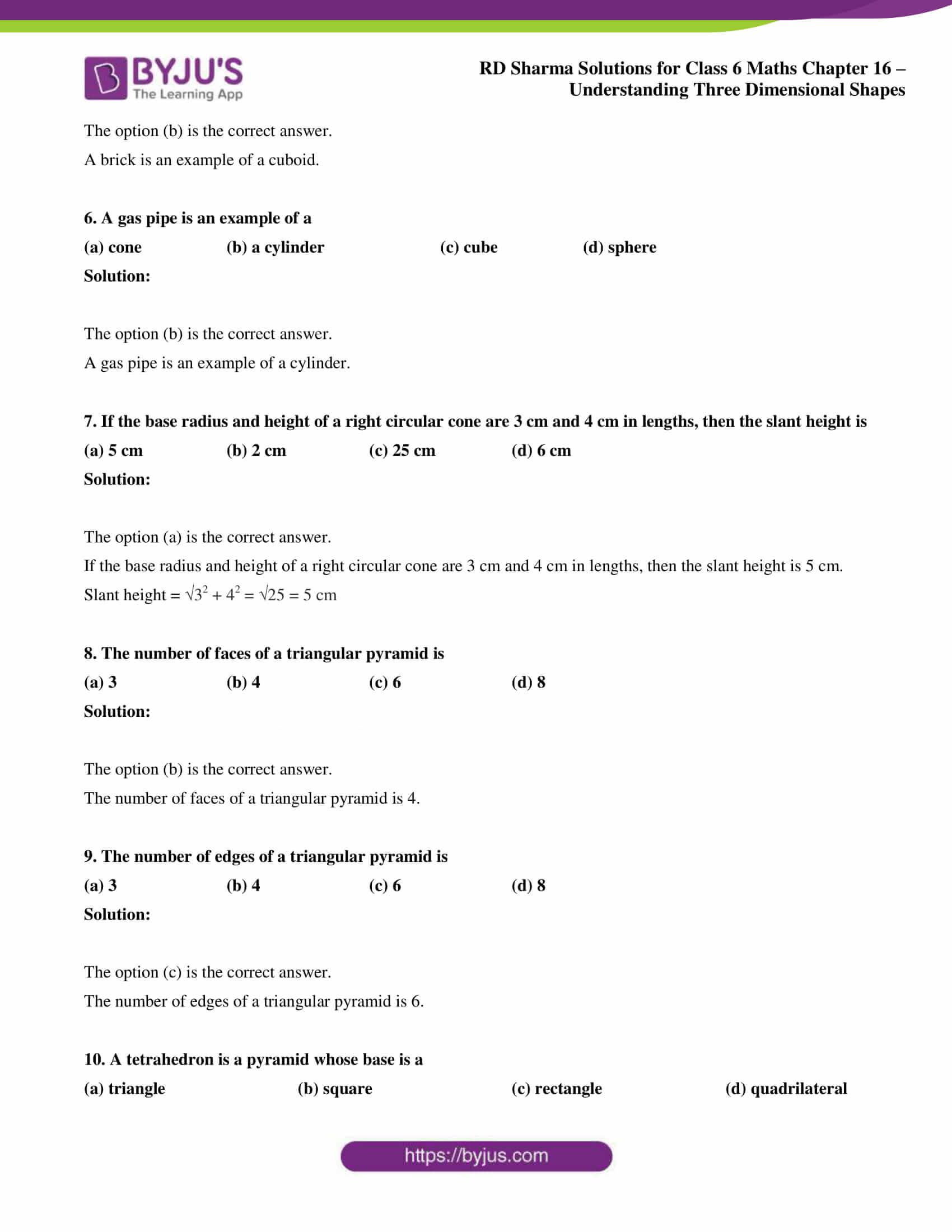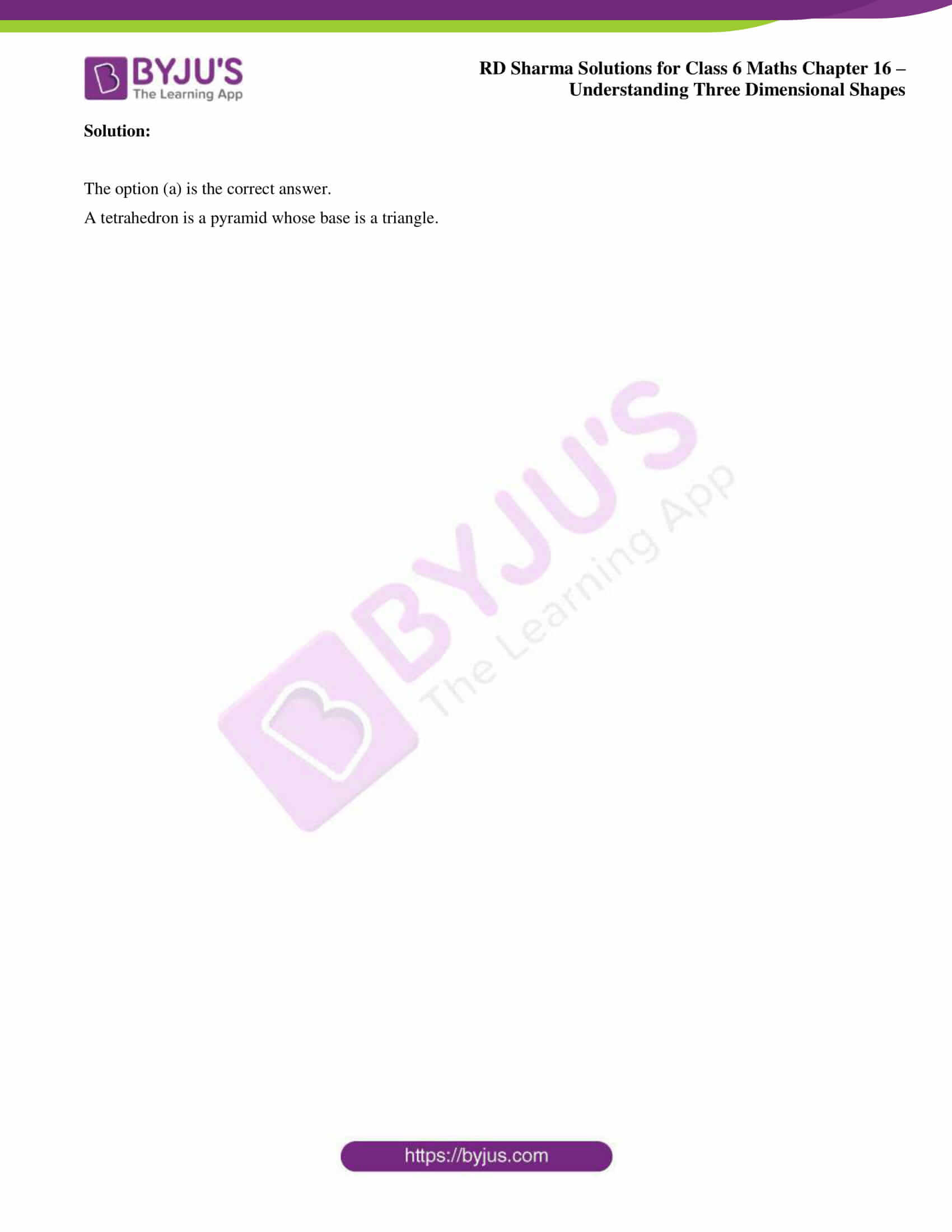# RD Sharma Solutions for Class 6 Maths Chapter 16: Understanding Three Dimensional Shapes Objective Type Questions

The objective type questions are present at the end of each chapter to help students self analyse their performance. The students can gain an overall idea about the concepts which are covered in this Chapter by answering the objective type questions. The solutions contain important formulas and shortcuts which are used in solving the problems to make it easier for the students during exam preparation. The students can access RD Sharma Solutions Class 6 Maths Chapter 16 Understanding Three Dimensional Shapes Objective Type Questions PDF for free from the links which are provided below.

## RD Sharma Solutions for Class 6 Maths Chapter 16: Understanding Three Dimensional Shapes Objective Type Questions Download PDF### Objective Type Questions page: 16.9

Mark the correct alternative in each of the following:

1. Total number of faces of a cuboid is

(a) 4 (b) 6 (c) 8 (d) 12

Solution:

The option (b) is the correct answer.

Total number of faces of a cuboid is 6.

2. Total number of edges of a cuboid is

(a) 4 (b) 6 (c) 8 (d) 12

Solution:

The option (d) is the correct answer.

Total number of edges of a cuboid is 12.

3. Number of vertices of a cuboid is

(a) 4 (b) 6 (c) 8 (d) 10

Solution:

The option (c) is the correct answer.

Number of vertices of a cuboid is 8.

4. Which one of the following is an example of a cuboid?

(a) a dice (b) a football (c) a gas pipe (d) an ice-cream cone

Solution:

The option (a) is the correct answer.

Example of a cuboid is a dice.

5. A brick is an example of a

(a) cube (b) cuboid (c) prism (d) cylinder

Solution:

The option (b) is the correct answer.

A brick is an example of a cuboid.

6. A gas pipe is an example of a

(a) cone (b) a cylinder (c) cube (d) sphere

Solution:

The option (b) is the correct answer.

A gas pipe is an example of a cylinder.

7. If the base radius and height of a right circular cone are 3 cm and 4 cm in lengths, then the slant height is

(a) 5 cm (b) 2 cm (c) 25 cm (d) 6 cm

Solution:

The option (a) is the correct answer.

If the base radius and height of a right circular cone are 3 cm and 4 cm in lengths, then the slant height is 5 cm.

Slant height = √32 + 42 = √25 = 5 cm

8. The number of faces of a triangular pyramid is

(a) 3 (b) 4 (c) 6 (d) 8

Solution:

The option (b) is the correct answer.

The number of faces of a triangular pyramid is 4.

9. The number of edges of a triangular pyramid is

(a) 3 (b) 4 (c) 6 (d) 8

Solution:

The option (c) is the correct answer.

The number of edges of a triangular pyramid is 6.

10. A tetrahedron is a pyramid whose base is a

(a) triangle (b) square (c) rectangle (d) quadrilateral

Solution:

The option (a) is the correct answer.

A tetrahedron is a pyramid whose base is a triangle.